# How to Create User Defined Functions in Oracle

## How to Create User-Defined Functions in Oracle

In this article, I am going to discuss How to Create User Defined Functions in Oracle with Examples. Please read our previous article where we discussed the basic concepts of User Defined Functions in Oracle.

##### Creating User-Defined Function in Oracle

Until now we understood what is meaning of function and the differences between a function and the procedures. Now, we will try to learn about how to create a function.Generally, we create the function using SQL Plus or SQL Developer. Once the code for the function is created. We will try to compile the function. If we find any errors we will check or compile the errors using SQL Developer or we can see the error using the command “show errors” and we can also check the errors in the table user_Errors, all_errors, dba_errors.

If the function is created without any errors, then the function can be invoked at any time in the database. Let’s now check the function creation with an example.

##### Example to Understand User-Defined Function in Oracle:

Create a function to return the salary for an employee. We need one parameter (in) number (employee_id). The return value should also be a number because it is salary. Please execute the below SQL Statement to create the function in the Oracle database.

```create or replace function get_sal
(p_emp_id number)
return number
is
v_sal number;
begin
select salary into v_sal
from employees
where employee_id=p_emp_id;

return v_sal;
end;
```

This is the statement for create function. We have created the function name with get_sal. This function takes an in parameter p_emp_id where the datatype is a number. This function also returns a number which is v_sal and the datatype is a number.

The PL/SQL block here indicates that we are giving the employee_id using the in-parameter p_emp_id and the PL/SQL block retrieves the salary of the given employee_id in the table employees. The salary will be stored in the parameter v_sal. The parameter v_sal will be returned to the function. The parameter will display the output to the user i.e. the salary using the v_sal parameter. Let’s go ahead and compile this function.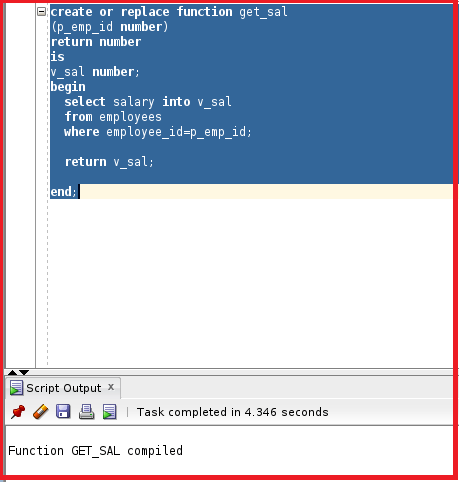The function is now compiled. Let’s go ahead and invoke the function. There are several methods to invoke a function.

#### Methods to Invoke a Function in Oracle:

Let us understand the different methods available in Oracle to Invoke User Defined Functions.

##### Method 1: Invoke a part of the Expression.
```declare
v_sal number;
begin
v_sal:=get_sal(100);
dbms_output.put_line (v_sal);
end;
```

We can invoke a function as a part of the expression. We have declared a variable v_sal with the datatype number. We have assigned that variable to the function get_sal. The get_sal will return a value and the value will be stored in the variable v_sal.

We have given the in parameter as 100. So, the 100 will be assigned to p_emp_id as it is employee_id. The PL/SQL block will select the table employees with the employee_id as 100 and will return a value of salary to the variable v_sal. The v_sal will return a value to the function. The returned value will be saved in the v_sal variable which is invoked as a part of the expression. We can use dbms_output to display the v_sal parameter. Let’s go ahead and execute the PL/SQL block.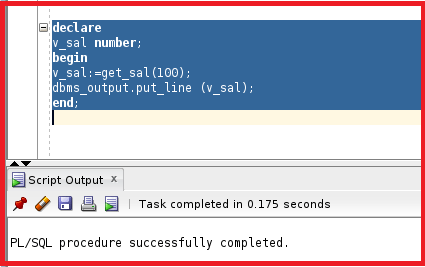So, the PL/SQL block is successfully compiled. Let’s check DBMS_OUTPUT as we have mentioned the DBMS_OUTPUT.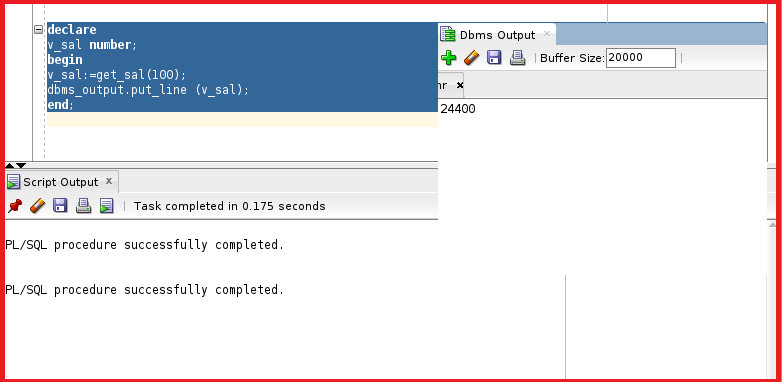We can see the DBMS Output is 24200 which is the salary of emp_id 100.

##### Method 2: Invoke Function as a Parameter Value.

Another way to use the function as a parameter value. In the DBMS_OUTPUT.PUT_LINE we can invoke the function and the value returned by the function will be displayed in the DBMS_OUTPUT.

```begin
dbms_output.put_line (get_sal(100));
end;
```

Let’s try to execute this.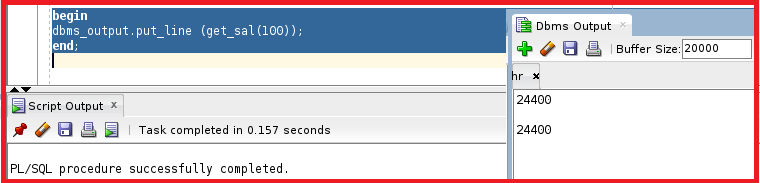We can see this displayed the same salary as the previous method.

##### Method 3: Invoke Function in the Execute Statement.

We can also execute because DBMS_OUTPUT is a package and PUT_LINE is the procedure. We will understand more about the package in the upcoming articles.

execute dbms_output.put_line(get_sal(100));

Let’s try to execute this.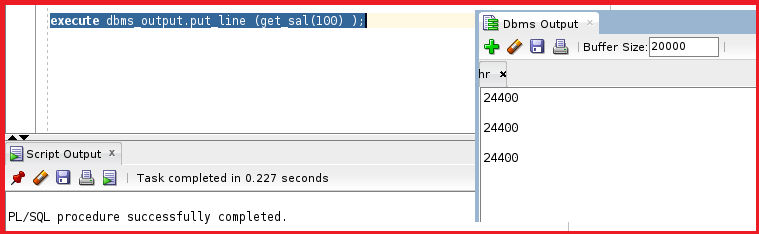We got the same result as the previous methods.

##### Method 4: Invoke Function By Defining a Bind Variable.

Let’s define a bind variable b_salary and assign the variable to the output of the function. So, the value returned by the function will be assigned to the bind variable and we can use the bind variable to print the value.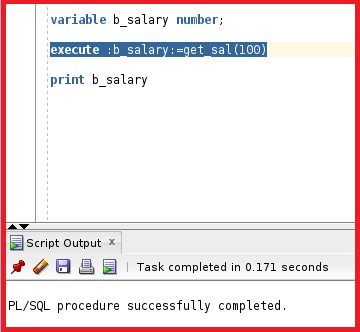So, we have created the bind variable b_salary and executed calling the function get_sal where the out value of the function is assigned to the variable b_salary. Now, let’s try to print the variable b_salary.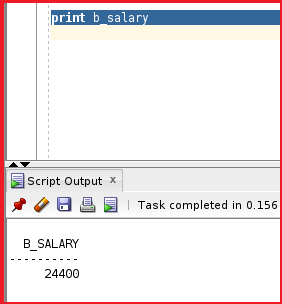So, we got the same salary as the previous methods.

##### Method 5: Invoke Function as a part of the Select Statement

We can invoke a function in the select statement.

select get_sal(100) from dual;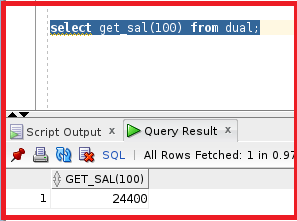So, we can able to call the function in the select statement as well. Let’s try another method.

Select employee_id, first_name, get_sal(employee_id) from employees Where department_id=20;

So, we fetching the salary of all employees whose department_id is 20. Let’s execute this statement and see the output.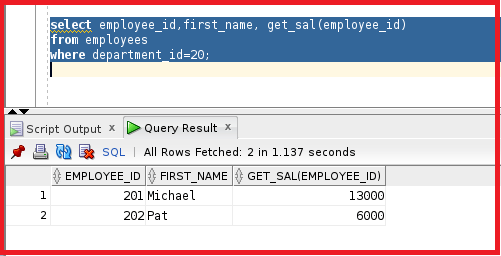So, this method is not good practice because the salary column is already present inside the table employees. By using the function in this select statement the function will be executed for each row. It means the function will take emp_id as 1 to 200. The function will be executed 200 times which is not a good sign.

##### How to find a function in user_objects?

The function will be in a table called user_objects. We can get the details of the function by using the table user_objects.

select * from user_objects where object_name=’GET_SAL’;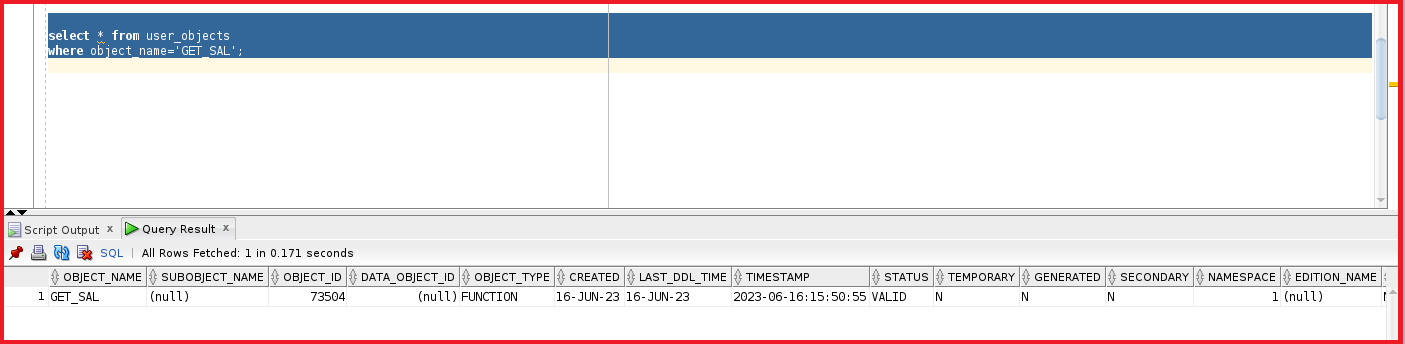We can see the object_name as GET_SAL and the object_type is a function and the status is Valid. We can also SQL code of the function using the view user_Source.

select line, text from user_source where name=’GET_SAL’;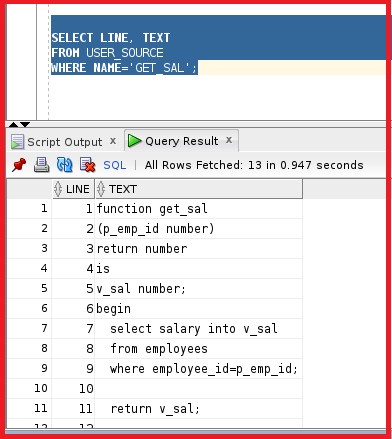We can see the SQL code used to create the function get_sal.

##### No_data_found in Oracle:

Let’s see the code of the function that we have created.

```create or replace function get_sal
(p_emp_id number)
return number
is
v_sal number;
begin
select salary into v_sal
from employees
where employee_id=p_emp_id;
return v_sal;
end;
```

The code for this function doesn’t contain an exception section. Let’s try to execute this function using method 2 and see the output once.

```begin
dbms_output.put_line (get_sal(100));
end;
```We can see the result. Now, let’s do the same example and give the employee_id as out of scope. Let’s try to give 9999. Actually, we don’t have an employee with employee_id 9999. Let’s try to execute this statement and see if we get any exceptions.

```begin
dbms_output.put_line (get_sal(100));
end;
```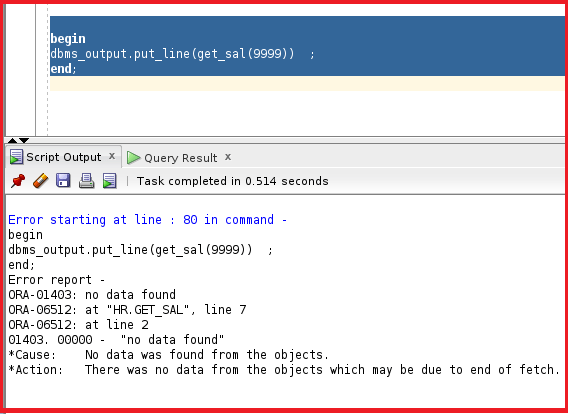So, we can the output as no data found. When we try to execute this 9999 from a select statement, we may get null. Let’s try to execute that as well.

Select get_sal(9999) from dual;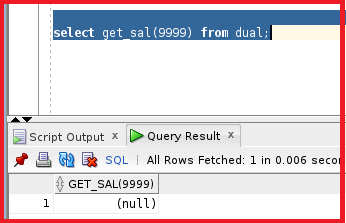We can see null. So, if the function does not contain an exception we will get no_data_found. Let’s try to create the function with an exception.

```create or replace function get_sal
(p_emp_id number)
return number
is
v_sal number;
begin
select salary into v_sal
from employees
where employee_id=p_emp_id;

return v_sal;
exception
when no_data_found then
return -1;

end;
```

In the exception section, we have mentioned that if no_data_found then we can return it with -1. The return in exception should be of the same data type as the return in the function. Let’s try to compile the function.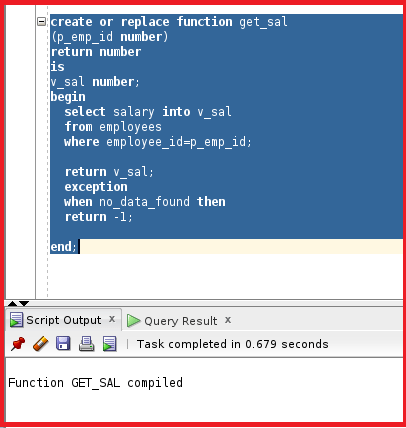So, the function is now compiled. Let’s now try to check the function using the 9999 employee_id

select get_Sal(9999) from dual;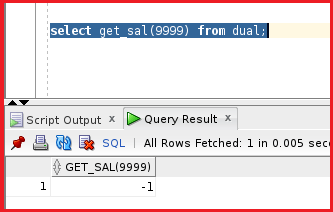We got the result as excepted. So, the exception is running now.

Note: If the function does not contain exceptions, we get the error. But if the function contains exceptions, then we will get the exception that we have mentioned in the function.

In the next article, I am going to discuss the Advantages of User Defined Functions in Oracle with Examples. Here, in this article, I try to explain How to Create User Defined Functions in Oracle with Examples. I hope you enjoy this Creating User Defined Function in Oracle article.